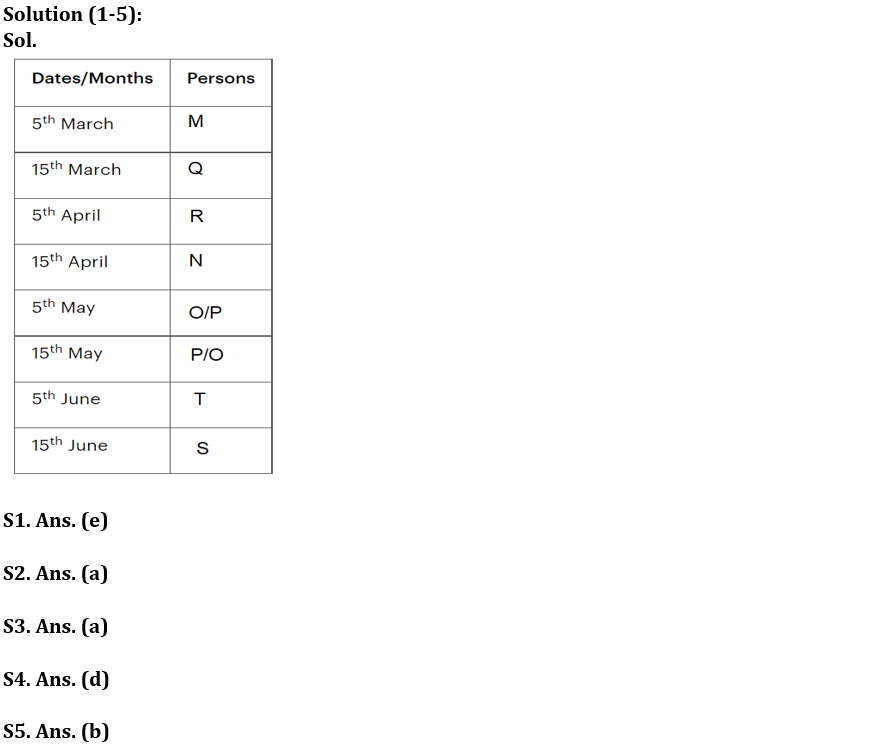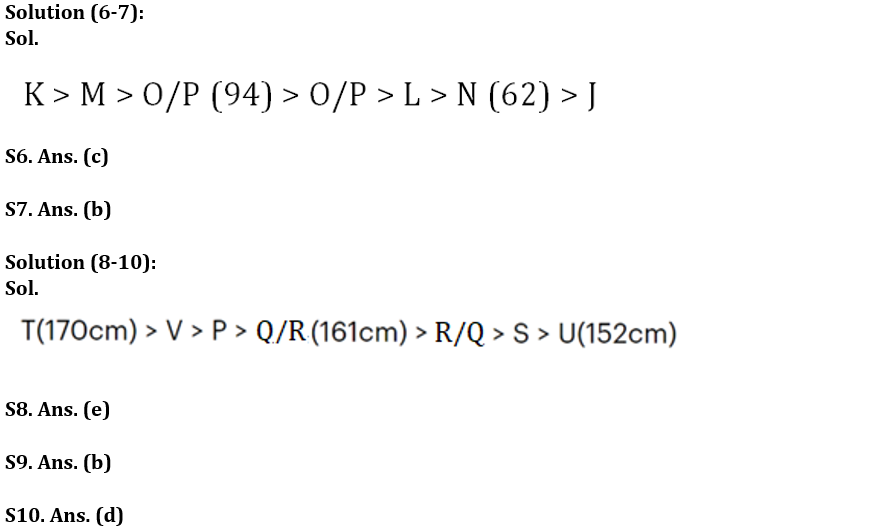Latest Banking jobs   »   Reasoning Quizzes Quiz For IRDA AM...

# Reasoning Quizzes Quiz For IRDA AM 2023-14th May

Directions (1-5): Answer the questions based on the information given below.
Eight persons M, N, O, P, Q, R, S and T have examinations on two different i.e., 5th or 15th of four different months (March, April, May, and June) of the same year but not necessarily in the same order.
M has exam on a prime numbered date. Three persons have exams between T and R. O and P has exam in the same month. Two persons have exams between M and N. Neither N nor R has exam in March and May. S and T have exam in the same month but after N.

Q1. Who among the following person has exam on 5th May?
(a) Q
(b) M
(c) O
(d) P
(e) Can’t be determined

Q2. How many persons have exam between M and R?
(a) One
(b) Two
(c) Three
(d) Four
(e) More than four

Q3. Q has the exam on which of the following date?
(a) 15th March
(b) 5th May
(c) 15th April
(d)15th June
(e) None of these

Q4. If P has exam immediately before T, then how many persons have exams before O?
(a) One
(b) Two
(c) Three
(d) Four
(e) More than four

Q5. Who among the following person has exam immediately after R?
(a) Q
(b) N
(c) O
(d) P
(e) None of these

Directions (6-7): Answer the questions based on the information given below:
Seven students J, K, L, M, N, O and P scored different marks in an examination. L scored more than only J and N. M scored less than only K. N does not score the least. The one who scored the third-highest marks, scored 94 marks and N scored 62 marks. The student who scored the maximum marks among all, scored 16 marks more than O’s marks.

Q6. If O scored 94 marks, then what will be sum of the marks scored by N and K?
(a) 175
(b) 176
(c) 172
(d) 185
(e) None of these

Q7. What may be possible marks scored by L, if O and P scored 94 and 80 marks respectively?
(a) 95
(b) 70
(c) 85
(d) 90
(e) 61

Directions (8-10): Answer the following question based on the information below.
Seven people, P, Q, R, S, T, U and V have different heights. P is taller than Q and R but shorter than T. S is taller than U but shorter than both R and Q. V is taller than P but is not the tallest. The height of the tallest person is 170cm. The height of the shortest person is 152 cm. The height of fourth tallest person is the average of tallest and shortest person.

Q8. How many people are taller than Q?
(a) One
(b) Three
(c) Two
(d) Four
(e) Either three or four

Q9. If R is shorter than Q, then what can be the possible height of R?
(a) 163cm
(b)157cm
(c) 164cm
(d) 174cm
(e) 160cm

Q10. Who among the following people is 2nd tallest?
(a) T
(b) P
(c) Either T or V
(d) V
(e) None of these

Solutions## FAQs

### How many sections are there in the IRDAI exam?

There are 4 sections in the IRDAI assistant manager online preliminary exam. They are Reasoning, English Language, General awareness, Quantitative aptitude.

#### Congratulations!Union Budget 2023-24: Free PDF